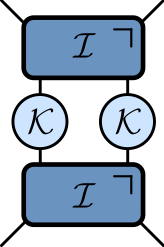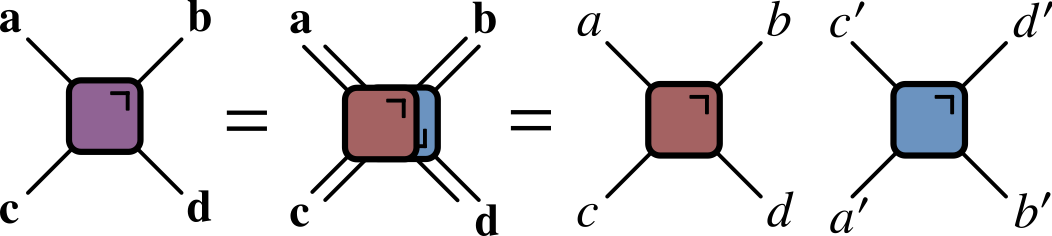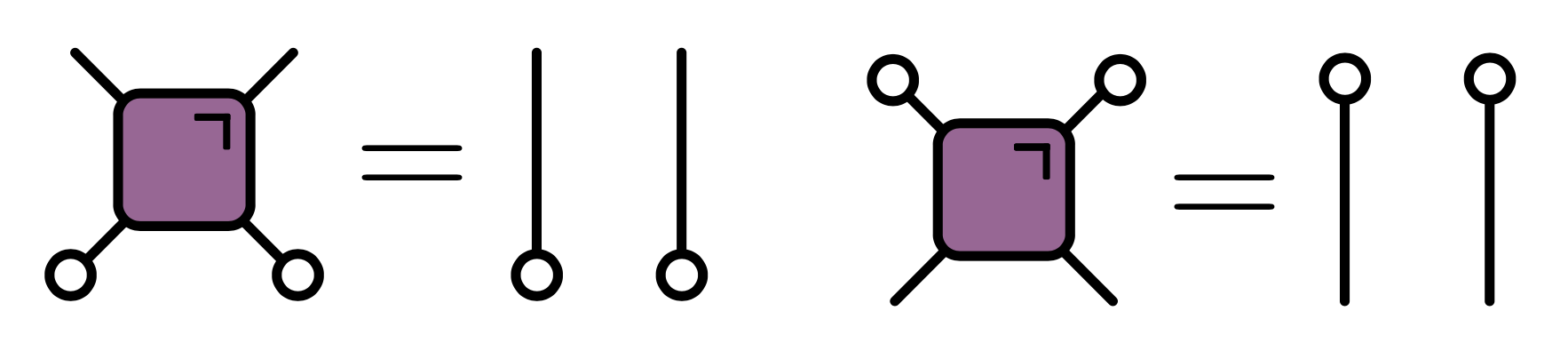# Quantum Circuits I

## Circuits as many-body dynamicsausten.uk/slides/quantum-circuits-1-icts for slides

## Outline

• This lecture

• What is a quantum circuit?
• What are the quantities of interest?
• Next lecture

• Some special kinds of circuits

## What is a quantum circuit?

• A way to describe operations on quantum state, usually consisting of several qubits (spin 1/2 subsystems with Hilbert space $\mathbb{C}^2$)

• Two quantum states $\ket{0}$ and $\ket{1}$ define the computational basis.• $f$ acts on top five qubits, then $g$ acts on lower seven

## Possible operations

1. $H$ (a Hadamard gate) is a single qubit unitary

2. Also two qubit unitary gates (CNOT here)

3. Measurements

## Why consider circuits?

• For this program: example of discrete time, many body dynamics

• Model of universal quantum computation

• How to generate an arbitrary quantum state
• One of several options e.g. measurement-based
• They exist! Companies (Google, IBM, etc.) have built platforms for gate-based QC

## Unitary circuits

• (Mostly) concerned with unitary circuits made from unitary gates

• Gate is $n$-qubit unitary $U_{s_1\ldots s_n,s’_1,\ldots, s’_n}$

$$\sum_{s_1'\ldots s_N'}U_{s_1\ldots s_n,s'_1,\ldots, s'_n} U^\dagger_{s'_1\ldots s'_n,s''_1,\ldots, s''_n}=\delta_{s_1,s_1''}\ldots \delta_{s_N,s_N''}$$

## Everything is a tensor

• State of $N$ qubits expressed in product basis

$$\ket{\Psi} = \sum_{s_{1:N}\in \{0,1\}^N} \Psi_{s_1\ldots s_N}\ket{s_1}_1\ket{s_2}_2\cdots \ket{s_N}_N$$

• Write $\ket{s_1}_1\ket{s_2}_2\cdots \ket{s_N}_N =\ket{s_1\cdots s_N}=\ket{s_{1:N}}$ for brevity

• Operator on $N$ qubits has matrix elements

$$\mathcal{O}_{s_{1:N},s'_{1:N}} = \bra{s_{1:N}}\mathcal{O}\ket{s'_{1:N}}$$

## Graphical notation

• A tensor is denoted by a blob with one leg for each index

• Connecting legs denotes contraction: summing over a shared index

## Unitary gates: one qubit

• Multiplication by a Pauli matrix: $X$, $Y$, or $Z$.

• General case $U = a_0\mathbb{1} + \mathbf{a}\cdot(X,Y,Z)$ with $|a_0|^2+|\mathbf{a}|^2=1$

• Other special cases used in quantum information e.g. Hadamard gate

$$H = \frac{1}{\sqrt{2}}\begin{pmatrix} 1 & 1 \\ 1 & -1 \end{pmatrix}$$

## Two qubits

• Work in basis $\ket{00}$, $\ket{01}$, $\ket{10}$, $\ket{11}$

• Simplest example is SWAP gate

$$\operatorname{SWAP}=\begin{pmatrix} 1 & 0 & 0 & 0 \\ 0 & 0 & 1 & 0 \\ 0 & 1 & 0 & 0 \\ 0 & 0 & 0 & 1 \end{pmatrix}$$.

$$\operatorname{SWAP}\ket{10} = \ket{01}$$

• SWAP takes product states to product states
• Slightly more complicated: square root of SWAP

$$\sqrt{\operatorname{SWAP}}=\begin{pmatrix} 1 & 0 & 0 & 0 \\ 0 & \frac{1}{2}(1+i) & \frac{1}{2}(1-i) & 0 \\ 0 & \frac{1}{2}(1-i) & \frac{1}{2}(1+i) & 0 \\ 0 & 0 & 0 & 1 \end{pmatrix}$$

• This generates entanglement: takes product state to non-product state

$$\sqrt{\operatorname{SWAP}}\ket{10} = \frac{1}{2}\left[(1+i)\ket{10}+(1-i)\ket{01}\right]$$

• $\sqrt{\operatorname{SWAP}}$ conserves number of 1s and 0s

• $\sqrt{\operatorname{SWAP}}$ together with arbitrary single qubit unitary operators form universal gate set that allows for universal quantum computation

## General two qubit unitary

• Any two-qubit unitary $U\in \mathcal{U(4)}$ can be written

$$U = e^{i \phi} (u_+ \otimes u_-) V[J_x, J_y, J_z] (v_- \otimes v_+)$$

• $u_{\pm}, v_{\pm} \in SU(2)$

\begin{align*} V[J_x, J_y, J_z] &= \exp \left[-i\left(J_x \sigma^x \otimes \sigma^x + J_y \sigma^y \otimes \sigma^y+ J_z \sigma^z \otimes \sigma^z\right)\right]\\ &= \begin{bmatrix} e^{-i J_z} \cos(J_-) & 0 & 0 & -i e^{-i J_z \sin(J_-)} \\ 0 & e^{iJ_z} \cos(J_+) & -ie^{i J_z} \sin(J_+) & 0 \\ 0 & -ie^{i J_z} \sin(J_+) & e^{iJ_z} \cos(J_+) & 0 \\ -i e^{-i J_z \sin(J_-)} & 0 & 0 & e^{-i J_z} \cos(J_-) \\ \end{bmatrix} \end{align*}

• 16 parameters!

## Time evolution

#### Single qubit gates

• Time evolution operator $U=\exp(-iHt)$

• If $H=\sum_j h_j$ a sum of single qubit terms

$$\mathcal{U} = \exp(-iHt) = \prod_j \exp(-ih_j) = \prod_j U_j$$ $$U_j=\mathbb{1}\otimes \ldots \otimes\mathbb{1} \otimes \overbrace{u_j}^{j\text{th factor}} \ldots \otimes\mathbb{1}$$

#### Two qubit gates

• Simplest example of two qubit interaction is exchange Hamiltonian

\begin{align*} h_{12} &= J\left[X\otimes X+Y\otimes Y+Z\otimes Z\right] =J\left[X_1X_2+Y_1Y_2 + Z_1Z_2\right]\\ &=2\operatorname{SWAP} - 1 \end{align*} $$U(J) = \exp(-ih_{12}) = e^{iJ}\left[\cos (2J) \mathbb{1} - i\sin (2J) \operatorname{SWAP}\right]$$

• Special cases

$$U(\pi/4)=\operatorname{SWAP}$$ $$U(\pi/8)=\sqrt{\operatorname{SWAP}}$$

• Fully rotationally invariant
• $H=\sum_{i,j} h_{i,j}$ a sum of two qubit terms with $[h_{i,j},h_{j,k}]\neq 0$
• $\mathcal{U}\neq \prod_{i,j} \exp(-ih_{i,j})$. More complicated!

• Suzuki–Trotter expansion: decompose $H=H_A + H_B$

$$\mathcal{U} = \exp(-iH) = \left[\exp\left(-\frac{iH}{n}\right)\right]^n \sim \left[e^{-iH_A/n} e^{-iH_B/n}\right]^n$$

$$H = \sum_j h_{j,j+1}$$ $$H_A = \sum_j h_{2j, 2j+1}\qquad H_B = \sum_j h_{2j-1, 2j}$$ $$e^{-iH_A/n}=\prod_j U_{2j,2j+1}\qquad e^{-iH_B/n} = \prod_j U_{2j-1,2j}$$## Floquet theory: kicked Ising model

• Time dependent Hamiltonian with kicks at $t=0,1,2,\ldots$.

\begin{align*} H_{\text{KIM}}(t) = H_\text{I}[\mathbf{h}] + \sum_{n}\delta(t-n)H_\text{K}\\ H_\text{I}[\mathbf{h}]=\sum_{j=1}^L\left[J Z_j Z_{j+1} + h_j Z_j\right],\qquad H_\text{K} &= b\sum_{j=1}^L X_j, \end{align*}

• “Stroboscopic” form of $\mathcal{U}(t)=\mathcal{T}\exp\left[-i\int^t H_{\text{KIM}}(t’) dt’\right]$

\begin{aligned} \mathcal{U}(n_+) &= \left[\mathcal{U}(1_+)\right]^n,\qquad U(1_-) = K I_\mathbf{h}\\ I_\mathbf{h} &= e^{-iH_\text{I}[\mathbf{h}]}, \qquad K = e^{-iH_\text{K}} \end{aligned}

## KIM as a circuit\begin{aligned} \mathcal{K} &= \exp\left[-i b X\right]\\ \mathcal{I} &= \exp\left[-iJ Z_1 Z_2 -i \left(h_1 Z_1 + h_2 Z_2\right)/2\right] \end{aligned}

## Locality

• Locality a feature of real quantum computing architectures

## Computational complexity

• State is vector in $2^N$ dimensional space

• Updating involves acting with a unitary matrix

• Naive matrix-vector multiplication $O(\operatorname{dim}^2)=2^{2N}$

• Since gates gives sparse matrices update is $O(\operatorname{dim})=2^{N}$

• Still exponentially hard in the number of qubits

## Expectation values

• Evaluate $\bra{\Psi}\mathcal{O}\ket{\Psi}=\bra{\Psi_0}\mathcal{U}^\dagger\mathcal{O}\mathcal{U}\ket{\Psi_0}$ for local operator $\mathcal{O}$

• Simplest example: $X$, $Y$, or $Z$ for one qubit

• $\mathcal{U}$ is overall unitary operator describing whole circuit

## Unitarity in graphical notation

• Have to consider both unitaries and conjugates

• Introduce color-coded notation

• Condition of unitarity $U^\dagger U = U U^\dagger = \mathsf{1}$

$$\sum_{\alpha,\beta}U^{\vphantom{\dagger}}_{a,b,\alpha,\beta} U^\dagger_{\alpha,\beta,c,d}=\delta_{ac}\delta_{bd}$$

• Unitarity of two qubit gate expressed as
• Much better!

## Using unitarity

• $\bra{\Psi}\mathcal{O}\ket{\Psi}=\bra{\Psi_0}\mathcal{U}^\dagger\mathcal{O}\mathcal{U}\ket{\Psi_0}$ has diagrammatic representation
• (leave initial state $\ket{\Psi}$ unspecified for the moment)

## “Folded” representations

• Since every $U$ accompanied by $U^\dagger$, include both in single unit by “folding” one on top of the other• Lines correspond to two indices, and therefore $2^2=4$ dimensions

• Unitarity condition takes form:• In folded picture expectation value $\bra{\Psi}\mathcal{O}\ket{\Psi}$ looks like this
• “Light cone” emerges, reflecting region of circuit that affects expectation value

## Reduced density matrix

• Expectation value of operator in region $A$ can be computed from reduced density matrix $\rho_A$ for region $A$ $$\rho_A = \operatorname{tr}_{B}\left[\ket{\Psi}\bra{\Psi}\right]=\operatorname{tr}_{B}\left[\mathcal{U}\ket{\Psi_0}\bra{\Psi_0}\mathcal{U}^\dagger\right]$$ ($B$ is complement of $A$)

• Initial RDM of bottom part of wedge is all that matters!

## Quantifying entanglement

• RDM quantifies entanglement present in a quantum state describing a system composed of two subsystems A and B

• General state is vector $\in\mathcal{H}=\mathcal{H}_A\otimes\mathcal{H}_B$

• Write in terms of basis vectors $\ket{a}_A$ and $\ket{b}_B$ for A and B subsystems $$\ket{\Psi}_{AB} = \sum_{a=1}^{n_A}\sum_{b=1}^{n_B} \Psi_{ab}\ket{a}_A\ket{b}_B$$ $n_{A/B}=\operatorname{dim} \mathcal{H}_{A/B}$

• Now regard $\psi_{ab}$ as matrix and perform a singular value decomposition

• In new bases our state is

$$\ket{\Psi}_{AB} = \sum_{n=1}^{\min(n_A, n_B)} \sigma_n \ket{u_n}_A\otimes\ket{v_n}_B$$

• Note single sum, c.f. double sum earlier. This is Schmidt decomposition

• $\sigma_n$ are Schmidt coefficients (singular values of SVD)

• If only one nonzero singular value state we have product state, indicating no correlations between subsystems

• Simplest example displaying nontrivial entanglement is Bell state

$$\left|\Psi^{+}\right\rangle=\frac{1}{\sqrt{2}}\left(|0\rangle_A \otimes|1\rangle_B+|1\rangle_A \otimes|0\rangle_B\right)$$

• Schmidt coefficients both $\frac{1}{\sqrt{2}}$, indicating maximal entanglement

• Schmidt decomposition closely related to RDM $$\rho_A = \operatorname{tr}_B\left[\ket{\Psi}\bra{\Psi}\right] = \sum_n \sigma_n^2 \ket{u_n}\bra{u_n}$$

• Eigenvalues of RDM are $p_n=\sigma_n^2$

• One measure of entanglement is von Neumann entropy of $\rho_A$ (aka entanglement entropy)

$$S^{(\text{vN})}_A \equiv -\operatorname{tr}\left[\rho_A\log \rho_A\right]$$

• $S_A$ vanishes for product state, and is otherwise positive
• Rényi entropies provide more complete information $$S^{(\alpha)}_A = \frac{1}{1-\alpha}\log \text{tr}\left[\rho^n\right]=\frac{1}{1-\alpha}\sum_n p_n^\alpha$$ $S^{(\text{vN})}_A=\lim_{\alpha\to 1} S^{(\alpha)}_A$. $S^{(0)}_A$ is number of nonzero Schmidt coefficients (aka Schmidt rank) $$S^{(2)}_A = -\log \sum_n p_n^2 = -\log \gamma\tr \rho_A^2$$ where $\gamma\equiv \tr \rho_A^2$ is purity

## Toy model

• Circuit of SWAP gates
• Initial state is product of Bell states $$\ket{\Phi^+}_{2n, 2n+1} = \frac{1}{\sqrt{2}}\left[\ket{0}_{2n}\ket{0}_{2n+1}+ \ket{1}_{2n}\ket{1}_{2n+1}\right]$$ $$\operatorname{tr}_{2}\left[\ket{\Phi^+}_{12}\bra{\Phi^+}_{12}\right] = \frac{1}{2}\mathbb{1}_1$$ with entanglement entropy of one bit
• $\rho_A$ therefore has factor $\mathbb{1}_n$ for each site $n\in A$ with “partner” in $B$

• If both qubits of a Bell pair are at sites $n,m\in A$ they give a factor $\ket{\Phi^+}_{nm}\bra{\Phi^+}_{nm}$: a pure state

• Entanglement entropy has contributions from first case only

$$S_A = \min(4\lfloor t/2\rfloor, |A|) \text{ bits}$$

$$S_A = \min(4\lfloor t/2\rfloor, |A|) \text{ bits}$$

• After time $\sim |A|/2$ subsystem has thermalized.
• Ramp behaviour in many systems

• In noninteracting systems or integrable systems, often explained in terms of the causal propagation of (quasi-)particles:

• Toy model with SWAP gates is rather similar, with qubits playing the role of “noninteracting particles”

• This picture remains true in circuits where there is no quasiparticle interpretation (next lecture)

## Correlation functions

• Natural correlation function to consider is

$$c_{\alpha \beta}(x,t) = \frac{1}{2^N}\tr\left[\sigma_{\alpha}(x,t) \sigma_{\beta}(0,0) \right],\qquad \sigma_\alpha(x,t)=\mathcal{U}^\dagger(t)\sigma_\alpha(x)\mathcal{U}(t)$$

• Average overall all initial states uniformly

• This is “infinite temperature”, although temperature is not defined

## Graphical representation

$$c_{\alpha \beta}(x,t) = \frac{1}{2^N}\tr\left[\sigma_{\alpha}(x,t) \sigma_{\beta}(0,0) \right],\qquad \sigma_\alpha(x,t)=\mathcal{U}^\dagger(t)\sigma_\alpha(x)\mathcal{U}(t)$$

• When $|x|>t$ unitarity condition leads to removal of all $U$s and $U^\dagger$s $$c_{\alpha \beta}(x,t) = \frac{1}{4}\tr\left[\sigma_{\alpha}\right]\tr\left[\sigma_{\beta}\right]=0,$$ and correlations vanish (if operators are traceless)

• Correlations only nonzero inside “light cone”

• When $|x|=t$ remaining tensor network is particularly simple:
• Rewrite in several ways, including in folded representation:
• $q$ is local Hilbert space dimension ($q=2$ up to now)

• Normalization factor by comparing with $\sigma_\alpha=\sigma_\beta=\mathsf{1}$ (here $t=4$)

• Evaluate by iteratively applying operator map $\mathcal{M}_+$ or $\mathcal{M}_-$

\begin{align*} \langle \sigma_{\alpha}(t,t) \sigma_{\beta}(0,0) \rangle &= \tr \left[\sigma_{\beta}\mathcal{M}_{-}^t(\sigma_{\alpha})\right] / q \\ &= \tr \left[ \sigma_{\alpha}\mathcal{M}_{+}^{t}(\sigma_{\beta})\right] / q \end{align*}

• $\mathcal{M}_\pm$ are examples of quantum channels: completely positive trace preserving maps between spaces of operators

• $\mathcal{M}_\pm$ have the additional property of being unital: $\mathcal{M}_\pm(\mathsf{1})=\mathsf{1}$

## Typical behaviour of correlations

• Can evaluate correlation inside light cone
• “One step inside” involves quantum channel acting on two-site operators: a space of dimension $q^4$.

• Taking $s$ steps inside channel acting on $q^{2s}$-dimensional space

• General situation is really exponentially hard, as we’d expect

• How does a local operator “look” as it evolves in Heisenberg picture?

• $Z_n(t)=\mathcal{U}^\dagger(t)Z_n \mathcal{U}(t)$ appears in $\langle Z_n(t)Z_m(0) \rangle$, but this is only one “component” of $Z_n(t)$

• Any observable such as $Z_n(t)$ can be expressed as expansion $$Z_n(t)= \sum_{\mu_{1:N}=\{1,x,y,z\}^N} \mathcal{C}_{\mu_{1:N}}(t) \sigma_1^{\mu_1}\otimes\cdots \sigma_N^{\mu_N}$$ $$\mathcal{C}_{\mu_{1:N}}(0)=\begin{cases} 1 & \mu_j=z, \mu_k=1,\forall k\neq j \\ 0 & \text{otherwise} \end{cases}$$

$$Z_n(t)= \sum_{\mu_{1:N}=\{1,x,y,z\}^N} \mathcal{C}_{\mu_{1:N}}(t) \sigma_1^{\mu_1}\otimes\cdots \sigma_N^{\mu_N}$$

• Since $\tr\left[\sigma_\alpha\sigma_\beta\right]=2\delta_{\alpha\beta}$, can extract spin correlations from $\langle Z_j(t)Z_k(0)\rangle=C_{jk}(t) \equiv \mathcal{C}_{1\cdots \mu_k=z \cdots 1}(t)$
• This represents only one component of operator expansion
• Operator norm $\tr\left[Z_n^2(t)\right]=2$ is conserved under time evolution

• This implies the normalization

$$\sum_{\mu_{1:N}=\{1,x,y,z\}^N} \mathcal{C}^2_{\mu_{1:N}}(t) = \frac{1}{2^{N-1}}.$$

## Example: $SU(2)$ preserving gate

• Consider gate generated by exchange Hamiltonian: $$U_{j,j+1} = \cos\theta \mathbb{1}_{j,j+1} + i\sin\theta \operatorname{\mathsf{S}}_{j.j+1}$$ $\operatorname{\mathsf{S}}_{j,j+1}$ denotes $\operatorname{\mathsf{SWAP}}$ gate on sites $j$ and $j+1$

• Action of this gate on an operator is

$$\mathcal{O} \longrightarrow U^\dagger_{j,j+1}\mathcal{O}U_{j,j+1} = \cos^2\theta \mathcal{O} + \sin^2\theta \operatorname{\mathsf{S}}_{j.j+1}\mathcal{O} \operatorname{\mathsf{S}}_{j.j+1} \\ -i\sin\theta\cos\theta \left[\operatorname{\mathsf{S}}_{j.j+1}, \mathcal{O}\right]$$

## Ensemble of circuits

• Consider ensemble averaged quantities

• Take $\theta=\pm \theta_0$ with $p(\theta_0)-p(-\theta_0)\equiv \delta > 0$.

• Averaging the evolved operator gives

$$\overline{U^\dagger_{j,j+1}\mathcal{O}U_{j,j+1}} = \cos^2\theta_0 \, \mathcal{O} + \sin^2\theta_0 \, \mathsf{S}_{j.j+1}\mathcal{O} \mathsf{S}_{j.j+1} \\ +i\delta \sin\theta_0\cos\theta_0 \left[\mathsf{S}_{j.j+1}, \mathcal{O}\right]$$

• Interpretation

1. Operators on sites $j$ and $j+1$ switch with probability $\sin^2\theta_0$.
2. The asymmetry $\delta$ governs strength of “quantum” dynamics
• Continuous time limit (Claeys, Lamacraft & Herzog-Arbeitman (2022) $$\frac{d\bar{\mathcal{O}}}{dt} = \sum_j \left[iJ \left[\mathsf{S}_{j,j+1},\bar{\mathcal{O}}\right]+\left(\mathsf{S}_{j,j+1}\bar{\mathcal{O}}\mathsf{S}_{j,j+1}-\bar{\mathcal{O}}\right)\right]$$ where $J\propto \delta$. Computing commutator:

\begin{align*} i[\mathsf{S},\sigma^a\otimes 1]&=-\epsilon^{abc}\sigma^b\otimes\sigma^c\nonumber\\ i[\mathsf{S},1\otimes \sigma^a]&=\epsilon^{abc}\sigma^b\otimes\sigma^c\nonumber\\ i[\mathsf{S},\sigma^a\otimes \sigma^b]&=\epsilon^{abc}\left(\sigma^c\otimes 1- 1\otimes \sigma^c\right). \end{align*}

• Describes operator “splitting” ($1\to 2$) and “merging” ($2\to 1$)

## $J=0$ limit

$$\frac{d\bar{\mathcal{O}}}{dt} = \sum_j \left[iJ \left[\mathsf{S}_{j,j+1},\bar{\mathcal{O}}\right]+\left(\mathsf{S}_{j,j+1}\bar{\mathcal{O}}\mathsf{S}_{j,j+1}-\bar{\mathcal{O}}\right)\right]$$

• No splitting and merging and terms

• In single operator sector define $\mathcal{C}^a_{0\cdots \mu_k=a\cdots 0}\equiv C^a_k$ $$\partial_t C^a_k = C^a_{k+1} + C^a_{k-1} - 2 C^a_k\equiv \Delta_k C^a_k,$$ diffusion of single $\sigma^a$ ($\Delta_k$ is 1D discrete Laplacian)

## $J\neq 0$

$$\partial_t \mathcal{C}_{\mu_{1:N}} = \sum_j \left[J\epsilon_{\alpha\beta \mu_j \mu_{j+1}} \mathcal{C}_{\mu_1\cdots \alpha\beta \cdots \mu_N} + \mathcal{C}_{\mu_1\cdots \mu_{j+1}\mu_j \cdots \mu_N} - \mathcal{C}_{\mu_1\cdots \mu_{j}\mu_{j+1} \cdots \mu_N}\right]$$

• First term leads to single site operator spreading over many sites• Qualitative behaviour is known as operator spreading and is a generic feature of operator dynamics
• Spreading suppressed at $J=0$ because we considered average

• In any sample from our random circuit, single-site operator spreads to many sites

• Random signs of coeffcients $\mathcal{C}_{\mu_{1:N}}$ means most average to zero: only the single site contributions remain

• When $J\neq 0$ some contribution survives and this allows for a controlled expansion

• We’d like a measure that is insensitive to these random signs